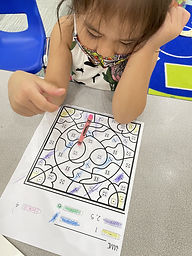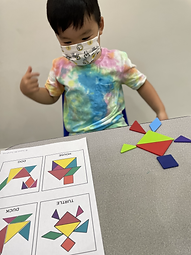## Ms. Kayla

### Target 1​

###### Lesson Type:

Continuation

Number Operation

:

Computation

Combine given quantities by using counting strategies.

###### 1:

Understand that combining numbers creates a larger quantity.

###### 2:

Represent “putting together” with objects, fingers, drawings, etc.

Pre-K

###### Vocabulary:

Activities:

1. Students rolled two dice and combined the numbers on the die that created a larger quantity. Students were exposed to mathematical vocabulary such as add, combine, put together, and equal. Students were also shown the numerical representation for their equations.### Home Exploration

###### Guiding Questions:## Absent Students:

### Target 2

:

###### 1:

Use one-to-one correspondence when counting to connect one quantity with one number name.

###### 2:

Count objects to determine how many there are.

###### 3:

Recognize the quantities zero to three, without having to count.

Pre-K

###### Vocabulary:

Count, Number, Color

Activities:

1. Students were given a worksheet to identify quantities of 1-6. The numbers were color coded and students needed to count the number of dots to match the correct color. Students were encouraged to quickly recognize numbers 1-3, but at times needed to use one-to-one correspondence.### Home Exploration

###### Guiding Questions:### Target 3

:

###### 1:

Solve simple tangram puzzles by recognizing which spaces the seven given shapes can and cannot fit in the puzzle.

Pre-K

###### Vocabulary:

Tangram, Triangle, Square, Shape, Top, Bottom

Activities:

1. Students were given tangrams and pictures of real-life objects to construct. Students were encouraged to follow the diagram by identifying the shapes used to create a specific image. Some images were constructed with the quidance of the teacher. Directional words were also used to help students with placement of the tangrams.### Home Exploration